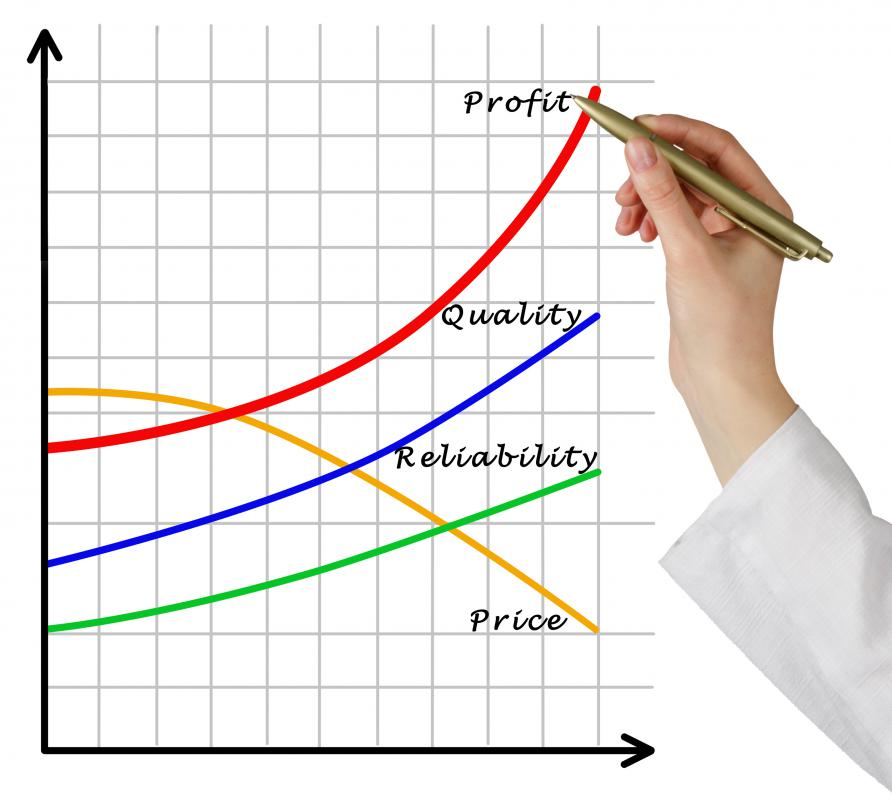# How do I Calculate Profit? (with pictures)

Patrick Roland
Patrick RolandRevenue minus expenses equals profit.

If you want to calculate profit, there is a surprisingly simple equation that helps you determine how much money a business earns after all its expenses. The equation itself is basic subtraction — revenue minus expenses — but determining total revenue and total expenses in order to perform this mathematical equation can be a challenge. With this answer, you can then calculate the profit percentage, because that is the number that many businesses use to gauge success.Shipping costs are considered expenses.

All you really need to calculate profit is a calculator and some figures. Subtracting the total expenses from the total revenue gives you the gross profit for any business or venture. However, finding these two numbers usually proves to be more work than the actual calculation.

When you calculate profit, know that total revenue is the amount of money a business brings in during a given period of time, usually during a quarter or a year. This refers to the money brought in from the sale of goods and services, but it also can include many other income streams. Many businesses place any savings into a bank account that collects interest, and if this is the case, it must be added to total revenue. Also, many companies that do not explicitly deal with real estate still own property and include any rent paid or sales of property in total revenue. There are many other possible sources of income, and all must be calculated as part of a business' revenue.

Similarly, a company's total expenses come from a variety of sources and are even more difficult to calculate than revenue. Expenses traditionally include the standard operating costs of a company, such as salaries, equipment, employee insurance, utilities and rent. This generally is everything for a service-providing business, but a goods-providing business has a massive amount of other expenses to consider. The cost of raw materials, the cost of any machinery or equipment used to create the final product, packaging and shipping all constitute costs that are added into total expenses.

With the total revenue and total expenses, it should be relatively easy to calculate profit by subtracting the expenses from the revenue. After you have the total profit, simply divide it by the total expenses to calculate the percentage of profit. For example, if your business had a total revenue of \$110,000 US Dollars (USD) and you subtracted \$100,000 USD of expenses, you would have earned \$10,000 USD worth of profit. For the percentage, simply divide the profit by the expenses and you have, in this case, a profit percentage of 10 percent.

## You might also Like## Discuss this Article

Forgot password?
###### Register:
•Revenue minus expenses equals profit.
•Shipping costs are considered expenses.